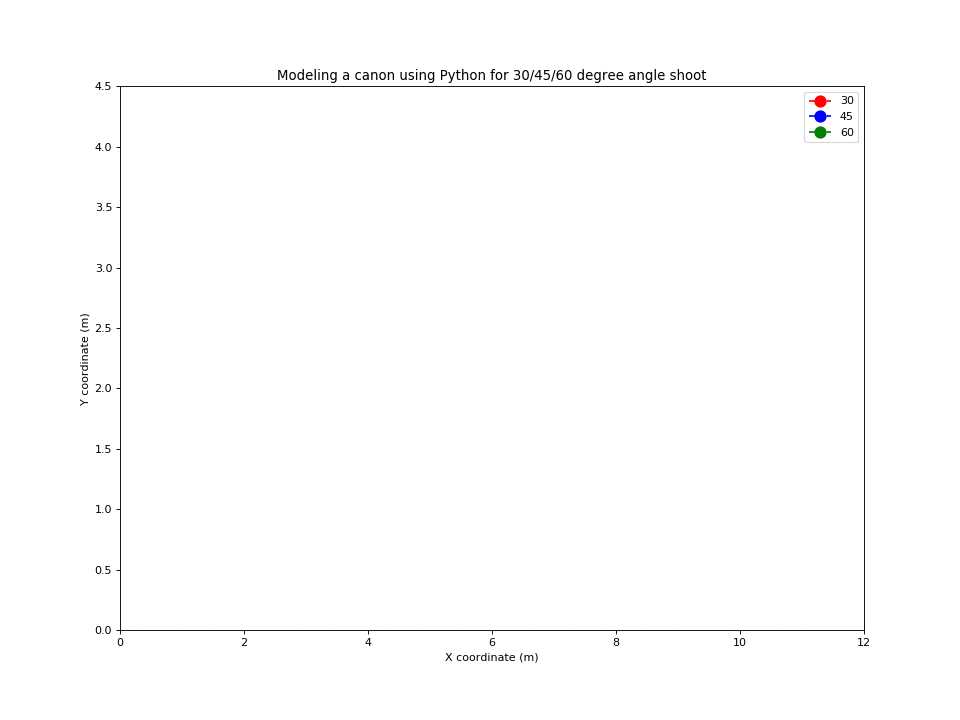Modeling a cannon using Python

Let us model a cannon using Python: given the same initial speed, how to hit a target in a given position with a cannon? Let the cannon be placed in the origin, i.e. its initial coordinate is (0,0) and the rest of parameters are:

$\begin{cases} x_0 = 0 \text{ meters}\\ y_0 = 0 \text{ meters}\\ t = 30 \text{ seconds} \\ v_0 = 10 m/s \\ g = -10 m/s^2 \\ \theta = 30,45,60 \end{cases}$

Suppose a cannon can fire a bullet with the initial velocity $$v_{0}$$ from the horizon. What is the best shooting angle $$\theta$$ that will fire the cannon bullet furthest ? As the bullet’s motion is determined by initial speed, angle and gravity. The two dimensional kinematic formula gives us all equations needed:

$\begin{cases} v_{x0} = v_0 \cos\theta \\ v_{y0} = v_0 \sin\theta \\ x_1 =x_0 + v_{x0} t \\ y_1 =y_0 + v_{y0} t + \frac{1}{2}gt ^2 \end{cases}$

Copy the code below, open the Python Playground, paste the code and run it.